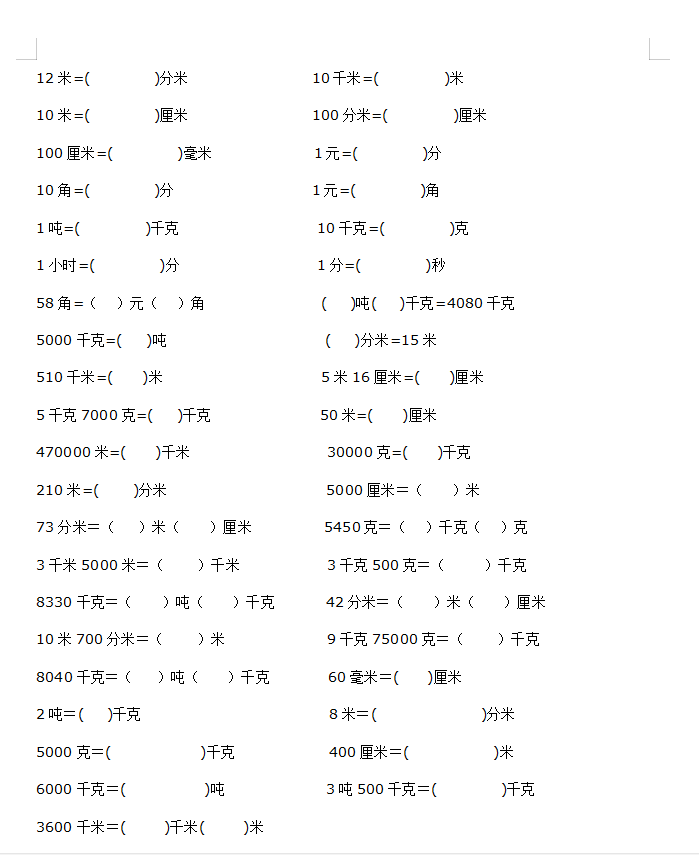12米=(            )分米                 10千米=(            )米

10米=(            )厘米                 100分米=(            )厘米

100厘米=(            )毫米              1元=(            )分

10角=(            )分                   1元=(            )角

1吨=(            )千克                   10千克=(            )克

1小时=(            )分                   1分=(            )秒

58角=（   ）元（   ）角                (    )吨(    )千克=4080千克

5000千克=(    )吨                      (    )分米=15米